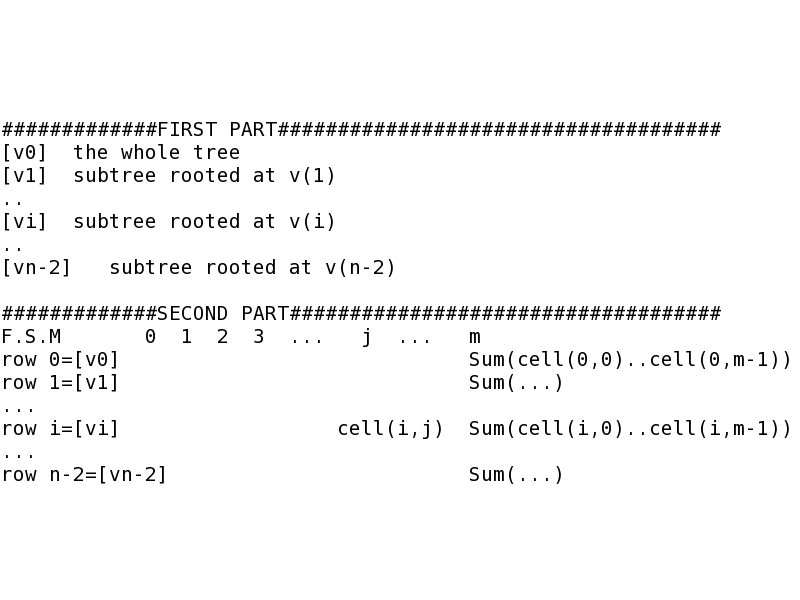# FitchAln

### INSTALLATION

• tar xvf FitchAln.tar

• The FitchAln software package is a free software developed in LINUX, compiled using g++ (4.4.1 or above) in LINUX operating system with the following command (Tests have been done in Fedora 11).

• make

• ### DESCRIPTION:

Given a newick binary tree T and its correpsonding multiple sequence alignemnt A of size n x m, where n is the number of leaf nodes in T and m is the length of the alignment, FitchAln generates a (n-1) x (m+1) Fitch score matrix representing the (Maximum Parsimony) number of mutations for each site for each internal node. We number the root of T as 0 and number all the remaining internal nodes in Broadth First Order. Each row i of the matrix represents an internal node numbered i. Therefore, row 0 represents the root, and there are (n-1) rows in total. Each column of the matrix, except the last column, represents a site of the sequence alignment. For all 0<=i<=n-2 and 0<=j<=m-1, cell(i,j) of the matrix shows the number of mutations for site j of the alignment for an internal node numbered i; while each cell in the last column gives the total numbers of mutations over all sites of the alignment for the internal node. That is, for all 0<=i<=n-2, when j equals to m, cell(i, m) equals to the sum of cell(i,0), ..., cell(i,m-1).

Input:
A newick binary tree T and a multiple sequence alignment A (of clustalw or fasta format). (T has n leaves in total, each leaf corresponds to exactly one sequence in A and each sequence has m characters, so that T has 2n-1 nodes in all and A has a size of n x m.)
Output:#### Format in FIRST PART:

There are n-1 rows in the first part in total. Each row has two columns, the first column is node and the second column is subtree.
node: [vi] (i is an integer ranged from 0 to n-2), represents an internal node of T numbered i. Note that the root of T is numbered 0 and all the remaining internal nodes are numbered in Breadth First Order. As T has n-1 internal nodes, these internal nodes are numbered from 0 to n-2.
subtree: a newick format subtree rooted at an internal node numbered i.

#### Format in SECOND PART

This is a (n-1) x (m+1) Fitch score matrix representing the (Maximum Parsimony) number of mutations for each site of the alignment for each internal node. Each row i of the matrix represents an internal node numbered i, and each column j, except the last one, represents site j of the alignment. For all 0<=i<=n-2 and 0<=j<=m-1, cell(i,j) of the matrix represents the Fitch score of site j of the alignment of the internal node numbered i; if j equals m, for all 0<=i<=n-2, cells(i,m) is the sum of cell(i,0),..,cell(i,m-1). Note that row 0 always represents the root (which is numbered 0).

### PROGRAM:

./FitchAln -i alnfile -f alnformat -t treefile -o outfile
• input_aln : an incoming multiple sequence alignment (MSA) file
• input_tree: an incoming (binary newick) phylogenetic tree, each exterior node of which represents a distinct sequence in the incoming MSA
• output: an outcoming result
• alnformat : format of input_aln, 0-clustalw, 1-fasta
• ### example:

./FitchAln -i example/T1.aln -f 0 -t example/T1.tree -o tmpout